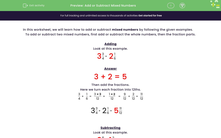# Add or Subtract Mixed Numbers

In this worksheet, students will add or subtract the given mixed numbers.Key stage:  KS 3

Curriculum topic:   Number

Curriculum subtopic:   Use Four Operations for All Numbers

Popular topics:   Percentages worksheets

Difficulty level:#### Worksheet Overview

In this activity, we will learn how to add or subtract mixed numbers by following the given examples.

To add or subtract two mixed numbers, first add or subtract the whole numbers, then the fraction parts.

Look at this example.

3
 3 4
+ 2
 1 6

3 + 2 = 5

Here we turn each fraction into 12ths.

 3 4
 1 6
 3 × 3 12
 1 × 2 12
 9 12
 2 12
 11 12

3
 3 4
+ 2
 1 6
= 5
 11 12

Subtracting

Look at this example.

3
 5 6
- 1
 2 9

3 - 1 = 2

Then subtract the fractions.

Here we turn each fraction into 18ths.

 5 6
-
 2 9
=
 5 × 3 18
-
 2 × 2 18
=
 15 18
-
 4 18
=
 11 18

3
 5 6
- 1
 2 9
= 2
 11 18

Remember to reduce or simplify fractions if you can.

So, for example, 6/8 should be reduced to 3/4 by dividing both the top and bottom numbers by 2.

Let's get started!

### What is EdPlace?

We're your National Curriculum aligned online education content provider helping each child succeed in English, maths and science from year 1 to GCSE. With an EdPlace account you’ll be able to track and measure progress, helping each child achieve their best. We build confidence and attainment by personalising each child’s learning at a level that suits them.

Get started